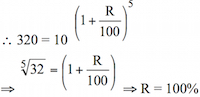# Sample Aptitude Questions of Mphasis

DIRECTIONS for the questions 1 to 10:  Solve the following question and mark the best possible option.
1. If a population of yeast cells grows from 10 to 320 in a period of five hours, what is the rate of growth?
1. It doubles its numbers every hour.
2. It triples its numbers every hour
3. It doubles its numbers every two hours.
4. It triples, its numbers every two hours
Cells grow from 10-320, so let the growth % be R per annum.So it doubles itself in every hour.
1. The number of red blood corpuscles in one cubic millimeter is about 5,000,000, and the number of white blood corpuscles in one cubic millimeter is about 8,000. What, then, is the ratio of white blood corpuscles to red blood corpuscles?
1. 1:625
2. 1:40
3. 4:10
4. 5:1250
8000 : 5,000,000 = 8 : 5000 =1:625.
2. Which of the following numbers can be divided evenly by 19?
1. 54
2. 63
3. 76
4. 82
76 comes in table of 19.
3. A rectangular tract of land measures 860 feet by 560 feet. Approximately how many acres is this? (one acre = 43,560 square feet)
1. 12.8 acres
2. 11.06 acres
3. 10.5 acres
4. 8.06 acres
Area of the rectangular tract = Length x Breadth = 860 x 560 Sq.ft. = {860x560}/43560 acres = 11.06 acres.
4. On a particular morning the temperature went up 1 ° every two hours. If the temperature was 53° at 5 A.M., at what time was it 57°?
1. 7 a.m.
2. 8 a.m
3. 12 p.m
4. 1 p.m
Difference in temperature= {57-53}= 4 degrees. 1 degrees rise happens in 2 hours , so 4 degrees rise would happen in 4 x 2 = 8 hrs, so it will be 8 hrs after 5 A.M. i.e. 1 P.M.
5. For health reasons, Amir wants to drink eight glasses of water a day. He has already had six glasses. What fraction is Amir left with?
1. 1/8
2. 1/6
3. 1/4
4. 1/3
The fraction of water he drank = 6/8= ¾, so fraction left = 1-3/4 = 1/4.
6. A movie is scheduled for two hours. The theatre advertisements are 3.8 minutes long. There are two previews; one is 4.6 minutes long, and the other is.2.9 minutes long. The rest of time is devoted to the feature film. How long is the feature film?
1. 108.7 minutes
2. 97.5 minutes
3. 118.98 minutes
4. 94.321 minutes
Total time apart from the feature film = { 3.8 + 4.6 + 2.9 }=11.3 minutes, therefore time for feature film = { 120-11.3}min= 108.7 min.
7. Twelve is 20% of what number?
1. 5
2. 20
3. 60
4. 240
20% of a no. is 12, so no.= 12/20%= 60.
8. The product of two and four more than three times a number is 20.What is the number?
1. 2
2. 16
3. 44
4. 87